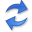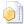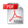Causal Inference by Compression

Abstract. Causal inference from observational data is one of the most fundamental problems in science. In general, the task is to tell whether it is more likely that $$X$$ caused $$Y$$, or vice versa, given only data over their joint distribution. In this paper we propose a general inference framework based on Kolmogorov complexity, as well as a practical and computable instantiation based on the Minimum Description Length (MDL) principle.

Simply put, we propose causal inference by compression. That is, we infer that $$X$$ is a likely cause of $$Y$$ if we can better compress the data by first encoding $$X$$, and then encoding $$Y$$ given $$X$$, than in the other direction. To show this works in practice, we propose Origo, an efficient method for inferring the causal direction from binary data. Origo employs the lossless Pack compressor (Tatti & Vreeken, 2008) and searches for that set of decision trees that encodes the data most succinctly. Importantly, it works directly on the data and does not require assumptions about neither distributions nor the type of causal relations.

To evaluate Origo in practice, we provide extensive experiments on synthetic, benchmark, and real-world data, including three case studies. Altogether the experiments show that Origo reliably infers the correct causal direction on a wide range of settings.

## Implementationthe Python source code (March 2017) by Kailash Budhathoki and Jilles Vreeken.

## Related PublicationsBudhathoki, K & Vreeken, J Origo: Causal Inference by Compression. Knowledge and Information Systems vol.56(2), pp 285-307, Springer, 2018. (IF 2.247)Budhathoki, K & Vreeken, J Causal Inference by Compression. In: Proceedings of the IEEE International Conference on Data Mining (ICDM'16), IEEE, 2016. (full paper, 8.5% acceptance rate; overall 19.6%)